The LOGISTIC Procedure
 SCORE Statement
SCORE <options> ;

The SCORE statement creates a data set that contains all the data in the DATA= data set together with posterior probabilities and, optionally, prediction confidence intervals. Fit statistics are displayed on request. If you have binary response data, the SCORE statement can be used to create a data set containing data for the ROC curve. You can specify several SCORE statements. FREQ, WEIGHT, and BY statements can be used with the SCORE statements. The SCORE statement is not available with the STRATA statement.

If a SCORE statement is specified in the same run as fitting the model, FORMAT statements should be specified after the SCORE statement in order for the formats to apply to all the DATA= and PRIOR= data sets in the SCORE statement.

See the section Scoring Data Sets for more information, and see Example 51.15 for an illustration of how to use this statement.

You can specify the following options:

ALPHA=number

specifies the significance levelfor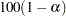% confidence intervals. By default, the value of number is equal to the ALPHA= option in the PROC LOGISTIC statement, or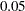if that option is not specified. This option has no effect unless the CLM option in the SCORE statement is requested.

CLM

outputs the Wald-test-based confidence limits for the predicted probabilities. This option is not available when the INMODEL= data set is created with the NOCOV option.

CUMULATIVE

outputs the cumulative predicted probabilities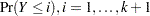, to the OUT= data set. This option is valid only when you have more than two response levels; otherwise, the option is ignored and a note is printed in the SAS log. These probabilities are named CP_level_i, where level_i is theth response level.

If the CLM option is also specified in the SCORE statement, then the Wald-based confidence limits for the cumulative predicted probabilities are also output. The confidence limits are named CLCL_level_i and CUCL_level_i. In particular, for the lowest response level, the cumulative values (CP, CLCL, CUCL) should be identical to the individual values (P, LCL, UCL), and for the highest response level CP=CLCL=CUCL=1.

DATA=SAS-data-set

names the SAS data set that you want to score. If you omit the DATA= option in the SCORE statement, then scoring is performed on the DATA= input data set in the PROC LOGISTIC statement, if specified; otherwise, the DATA=_LAST_ data set is used.

It is not necessary for the DATA= data set in the SCORE statement to contain the response variable unless you are specifying the FITSTAT or OUTROC= option.

Only those variables involved in the fitted model effects are required in the DATA= data set in the SCORE statement. For example, the following statements use forward selection to select effects:

```proc logistic data=Neuralgia outmodel=sasuser.Model;
class Treatment Sex;
model Pain(event='Yes')= Treatment|Sex Age
/ selection=forward sle=.01;
run;
```

Suppose Treatment and Age are the effects selected for the final model. You can score a data set that does not contain the variable Sex since the effect Sex is not in the model that the scoring is based on. For example, the following statements score the Neuralgia data set after dropping the Sex variable:

```proc logistic inmodel=sasuser.Model;
score data=Neuralgia(drop=Sex);
run;
```
FITSTAT

displays a table of fit statistics for the data set being scored. Four statistics are computed: total frequency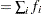, total weight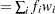, log likelihood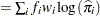, and misclassification rate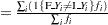, where the summations are over all observations in the data set being scored, and the values F_Y and I_Y are described in the section OUT= Output Data Set in a SCORE Statement.

OUT=SAS-data-set

names the SAS data set that contains the predicted information. If you omit the OUT= option, the output data set is created and given a default name by using the DATAconvention.

OUTROC=SAS-data-set

names the SAS data set that contains the ROC curve for the DATA= data set. The ROC curve is computed only for binary response data. See the section OUTROC= Output Data Set for the list of variables in this data set.

PRIOR=SAS-data-set

names the SAS data set that contains the priors of the response categories. The priors can be values proportional to the prior probabilities; thus, they do not necessarily sum to one. This data set should include a variable named _PRIOR_ that contains the prior probabilities. For events/trials MODEL syntax, this data set should also include an _OUTCOME_ variable that contains the values EVENT and NONEVENT; for single-trial MODEL syntax, this data set should include the response variable that contains the unformatted response categories. See Example 51.15 for an example.

PRIOREVENT=value

specifies the prior event probability for a binary response model. If both PRIOR= and PRIOREVENT= options are specified, the PRIOR= option takes precedence.

ROCEPS=value

specifies the criterion for grouping estimated event probabilities that are close to each other for the ROC curve. In each group, the difference between the largest and the smallest estimated event probability does not exceed the given value. The value must be between 0 and 1; the default value is the square root of the machine epsilon, which is about 1E–8 (in releases prior to 9.2, the default was 1E–4). The smallest estimated probability in each group serves as a cutpoint for predicting an event response. The ROCEPS= option has no effect if the OUTROC= option is not specified in the SCORE statement.Previous Page | Next Page | Top of Page